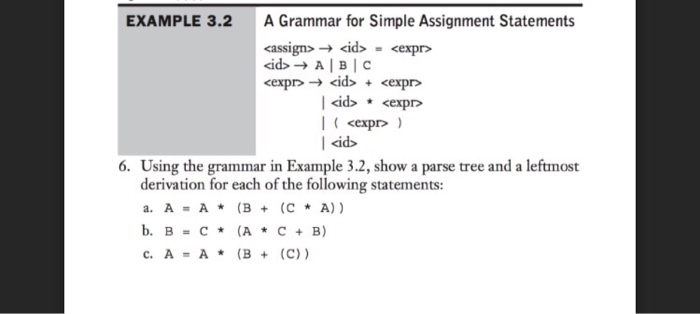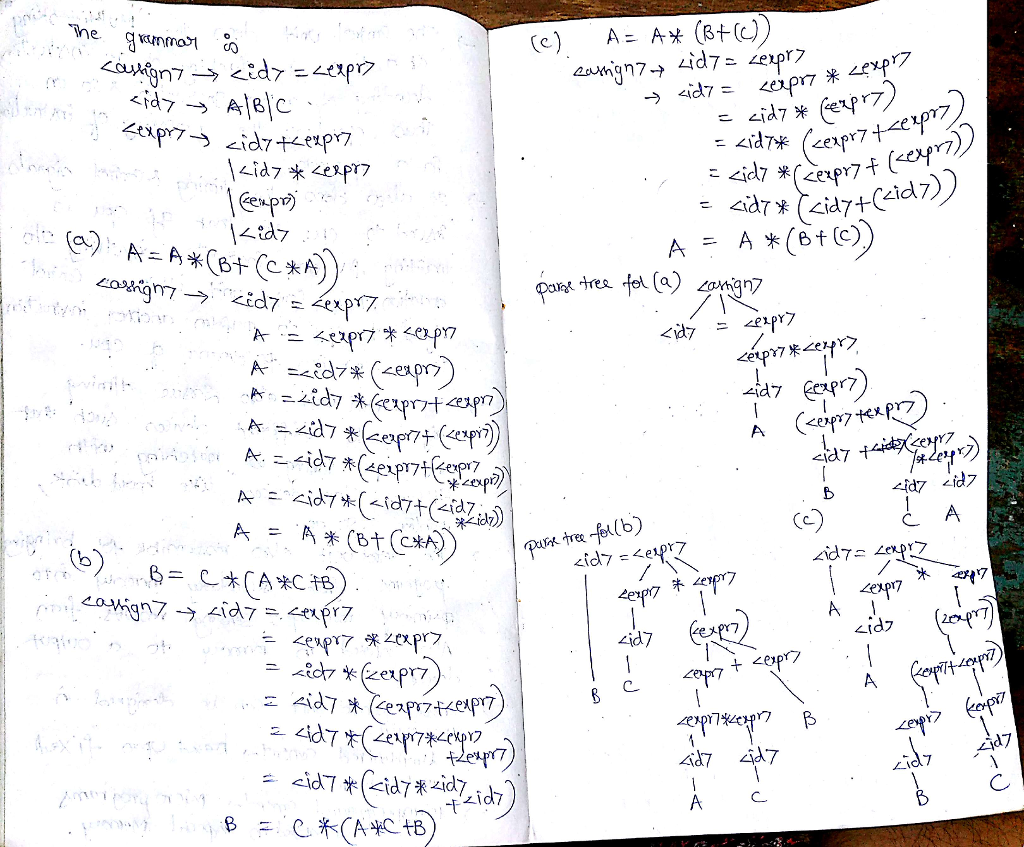# Question & Answer: A Grammar for Simple Assignment Statements rightarrow = rightarrow A | B | C rightarrow + | * | ( ) | Using the gramme…..A Grammar for Simple Assignment Statements rightarrow = rightarrow A | B | C rightarrow + | * | ( ) | Using the grammer in Example 3.2, show a parse tree and a leftmost derivation for each of the following statements: a. A = A * (B + (C * A)) b. B = C * (A * C + B) c. A = A * (B + (C))